Question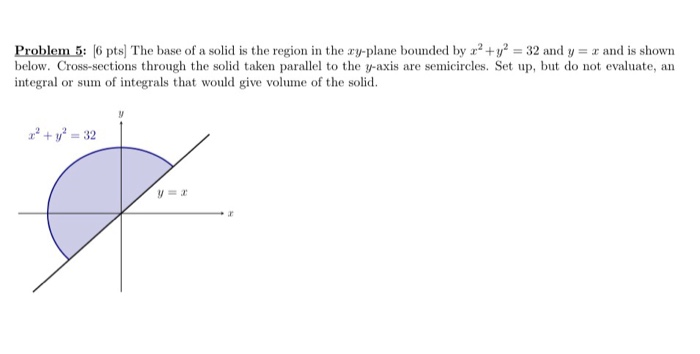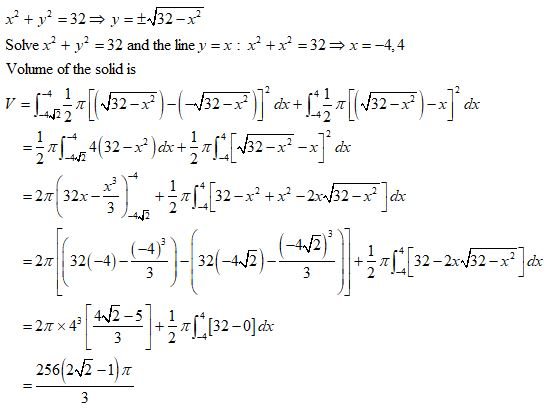#### Earn Coins

Coins can be redeemed for fabulous gifts.

Similar Homework Help Questions
• ### Problem 3 6 pts] The region R bounded by y V, y 0, and 4 is revolved about the line y3 Calculate the volume of the...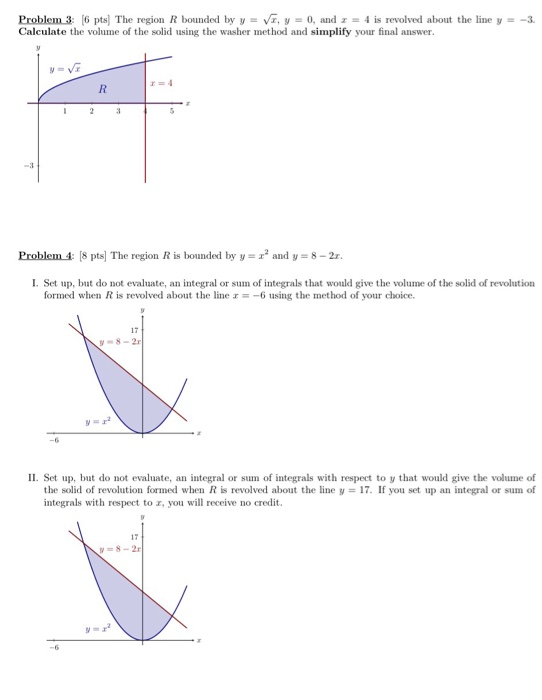Problem 3 6 pts] The region R bounded by y V, y 0, and 4 is revolved about the line y3 Calculate the volume of the solid using the washer method and simplify your final answer. -3 Problem 4: 8 pts] The region R is boud by y2 and y 8- 2 I. Set up, but do not evaluate, an integral or sum of integrals that would give the volume of the solid of revolution formed when R is revolved...

• ### The base of a solid is the region in the ry plane bounded by the curves...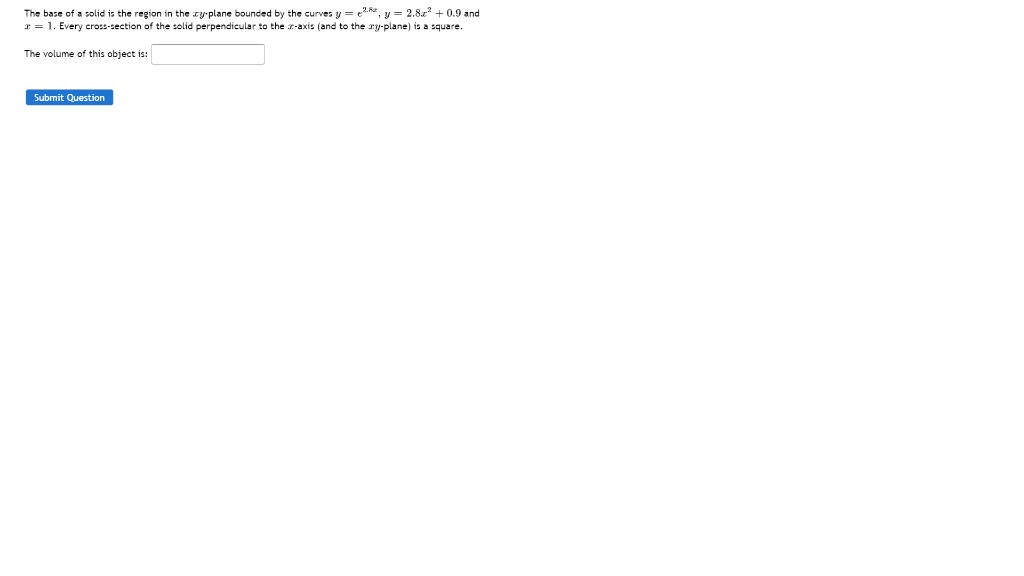The base of a solid is the region in the ry plane bounded by the curves y y =2.82 +0.9 and = 1. Every cross-section of the solid perpendicular to the r-axis (and to the ry.plane) is a square. The volume of this object is: Submit Question

• ### Problem 1 part II and Problem 2 part I and II Problem 1: (Short Answer) 6 pts] The region R is bounded by y 0, 0,...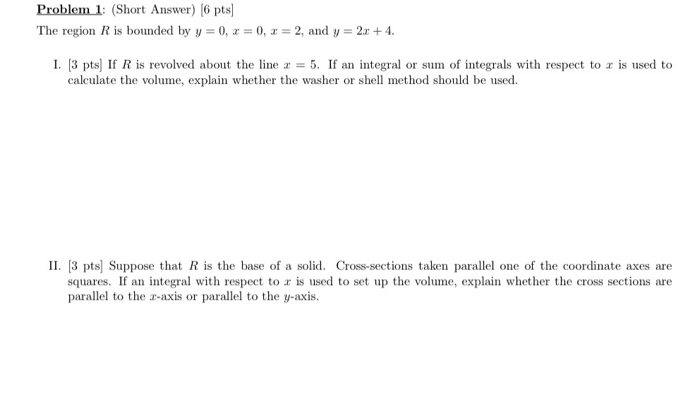Problem 1 part II and Problem 2 part I and II Problem 1: (Short Answer) 6 pts] The region R is bounded by y 0, 0, 2, and y- 2 4 1, 3 pts] If R is revolved about the line x = 5. If an integral or sunn of integrals with respect to z is used to calculate the volume, explain whether the washer or shell method should be used II. 3 pts) Suppose that R is the base...

• ### Consider a solid whose base is the region bounded by the curves y = (−x^2) + 3 and y = 2x − 5, wi...

Consider a solid whose base is the region bounded by the curves y = (−x^2) + 3 and y = 2x − 5, with cross-sections perpendicular to the y-axis that are squares. a) Sketch the base of this solid. b) Find a Riemann sum which approximates the volume of this solid. c) Write a definite integral that calculates this volume precisely. (Do not need to calculate the integral)

• ### only the sphere question the one above was inserted by mistake Consider the region bounded by...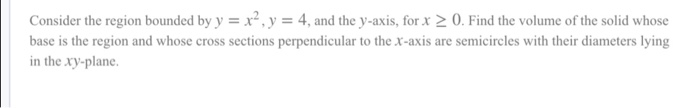only the sphere question the one above was inserted by mistake Consider the region bounded by y = x², y = 4, and the y-axis, for x > 0. Find the volume of the solid whose base is the region and whose cross sections perpendicular to the x-axis are semicircles with their diameters lying in the xy-plane. Give an equation representing the value of the stice you would use in a Riemann som representing the volume of the region. Then...

• ### The base of a solid is the region bounded by lines y = -1 + 2,...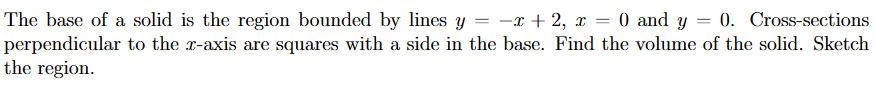The base of a solid is the region bounded by lines y = -1 + 2, x = 0 and y = 0. Cross-sections perpendicular to the z-axis are squares with a side in the base. Find the volume of the solid. Sketch the region.

• ### A solid has a base that is in the shape of the region bounded by the...

A solid has a base that is in the shape of the region bounded by the graphs of the following y=x^3 , y=2, and x=0 Cross sectional slices of this solid are semicircles that are perpendicular to both the base and the y-axis that have their diameters on that base. Compute the volume of this solid.

• ### Find the perimeter of the parametric curve given by cos3 t sin3t for 0sts2T (10) Find the volume of the solid whose base is the region bounded by the parabolas y2 and y 8- and whose cross-sections pe...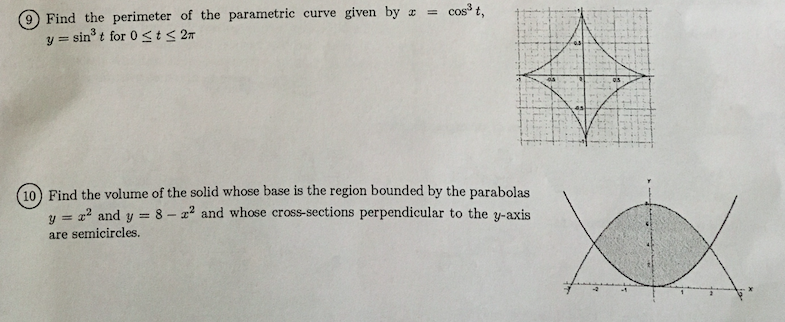Find the perimeter of the parametric curve given by cos3 t sin3t for 0sts2T (10) Find the volume of the solid whose base is the region bounded by the parabolas y2 and y 8- and whose cross-sections perpendicular to the y-axis are semicircles. Find the perimeter of the parametric curve given by cos3 t sin3t for 0sts2T (10) Find the volume of the solid whose base is the region bounded by the parabolas y2 and y 8- and whose cross-sections...

• ### 6. (12pts) Consider the solid that is above the xy-plane, bounded above by =/4-x-y and below by +y a. Sketch the so...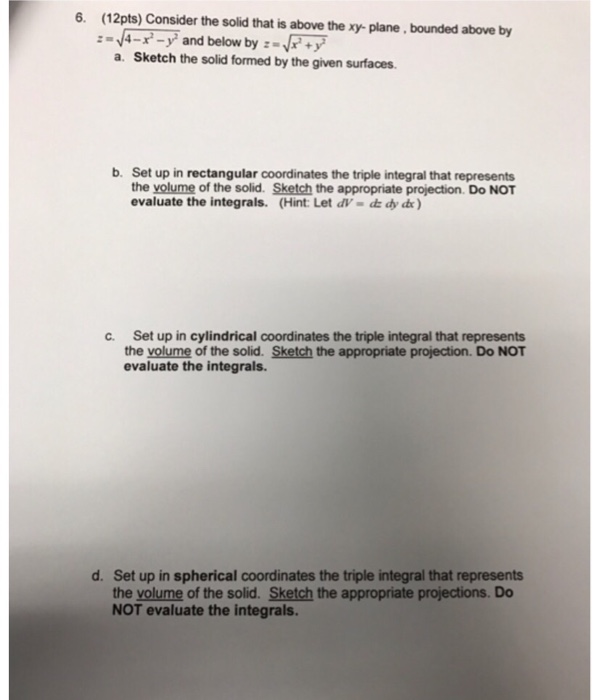6. (12pts) Consider the solid that is above the xy-plane, bounded above by =/4-x-y and below by +y a. Sketch the solid formed by the given surfaces b. Set up in rectangular coordinates the triple integral that represents the yolume of the solid. Sketch the appropriate projection. Do NOT evaluate the integrals. (Hint: Let dV- d dy de) c. Set up in cylindrical coordinates the triple integral that represents the volume of the solid. Sketch the appropriate projection. Do NOT...

• ### Problem 2 (1) Find the area enclosed by the curves y 2 and y-4z-z2 (2) Find the volume of the solid whose base is the triangular region with vertices(0, 0), (2, 0), and (0,1). Cross-sections perpendi...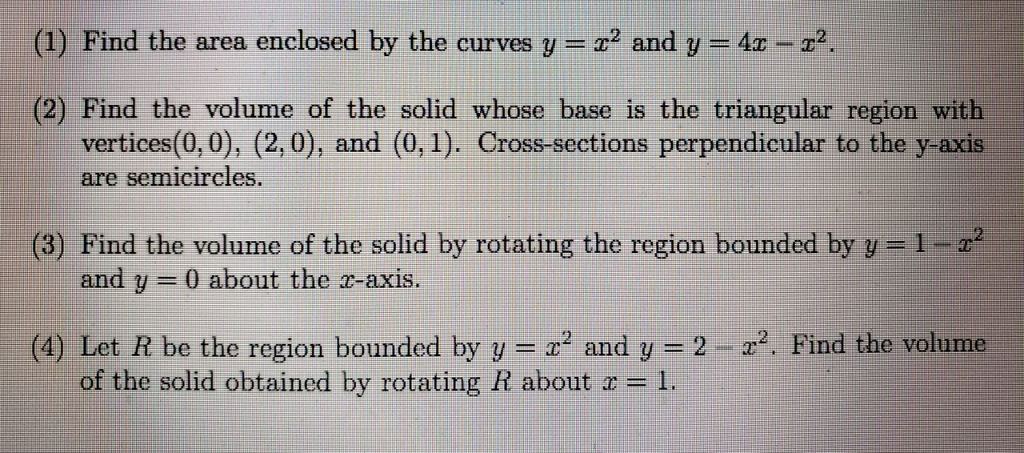Problem 2 (1) Find the area enclosed by the curves y 2 and y-4z-z2 (2) Find the volume of the solid whose base is the triangular region with vertices(0, 0), (2, 0), and (0,1). Cross-sections perpendicular to the y-axis semicircles. are (3) Find the volume of the solid by rotating the region bounded by y=1-z2 and y-0 about the r-axis. 2-z2. Find the volume (4) Let R be the region bounded by y--x2 and y of the solid obtained by...